Assertion & Reason Test: Triangles - 2

# Assertion & Reason Test: Triangles - 2 - Class 10

Test Description

## 10 Questions MCQ Test Mathematics (Maths) Class 10 - Assertion & Reason Test: Triangles - 2

Assertion & Reason Test: Triangles - 2 for Class 10 2023 is part of Mathematics (Maths) Class 10 preparation. The Assertion & Reason Test: Triangles - 2 questions and answers have been prepared according to the Class 10 exam syllabus.The Assertion & Reason Test: Triangles - 2 MCQs are made for Class 10 2023 Exam. Find important definitions, questions, notes, meanings, examples, exercises, MCQs and online tests for Assertion & Reason Test: Triangles - 2 below.
Solutions of Assertion & Reason Test: Triangles - 2 questions in English are available as part of our Mathematics (Maths) Class 10 for Class 10 & Assertion & Reason Test: Triangles - 2 solutions in Hindi for Mathematics (Maths) Class 10 course. Download more important topics, notes, lectures and mock test series for Class 10 Exam by signing up for free. Attempt Assertion & Reason Test: Triangles - 2 | 10 questions in 20 minutes | Mock test for Class 10 preparation | Free important questions MCQ to study Mathematics (Maths) Class 10 for Class 10 Exam | Download free PDF with solutions
 1 Crore+ students have signed up on EduRev. Have you?
Assertion & Reason Test: Triangles - 2 - Question 1

### Direction: In the following questions, A statement of Assertion (A) is followed by a statement of Reason (R). Mark the correct choice as.Assertion : Two similar triangle are always congruent.Reason : If the areas of two similar triangles are equal then the triangles are congruent.

Detailed Solution for Assertion & Reason Test: Triangles - 2 - Question 1
Two similar triangles are not congruent generally.

So, A is incorrect but R is correct.

Assertion & Reason Test: Triangles - 2 - Question 2

### Direction: In the following questions, A statement of Assertion (A) is followed by a statement of Reason (R). Mark the correct choice as.Assertion : ABC is an isosceles triangle right angled at C then AB2 = 2AC2.Reason : If in a triangle, square of one side is equal to the sum of the squares of the other two sides, then the angle opposite the first side is a right angle.

Detailed Solution for Assertion & Reason Test: Triangles - 2 - Question 2
We know that If in a triangle, square of one side is equal to the sum of the squares of the other two sides, then the angle opposite the first side is a right angle. This is converse of Pythagoras theorem.

So, Reason is correct By

Pythagoras theorem, we have AB2 = AC2 + BC2

= AC2 + AC2 [∵ AC = BC Given]

⇒ AB2 = 2AC2

So, Assertion is also correct.

But reason (R) is not the correct explanation of assertion (A).

Assertion & Reason Test: Triangles - 2 - Question 3

### Direction: In the following questions, A statement of Assertion (A) is followed by a statement of Reason (R). Mark the correct choice as.Assertion : ΔABC is an isosceles triangle right angled of C , then AB2 = 2AC2.Reason : In right ΔABC , right angled at B, AC2 =AB2 +BC2.

Detailed Solution for Assertion & Reason Test: Triangles - 2 - Question 3
In an isosceles ΔABC , right angled at C is

AB2 = AC2 + BC2

AB2 = AC2 + AC2

AB2 = 2AC2 (AC = BC)

So, both A and R are correct and R explains A.

Assertion & Reason Test: Triangles - 2 - Question 4

Direction: In the following questions, A statement of Assertion (A) is followed by a statement of Reason (R). Mark the correct choice as.

Assertion : ABC is an isosceles triangle with AC = BC. If AB2 = 2 AC2, then ΔABC is a right triangle.

Reason : If in a triangle, square of one side is equal to the sum of the squares of the other two sides, then the angle opposite the first side is a right angle.

Detailed Solution for Assertion & Reason Test: Triangles - 2 - Question 4
We know that If in a triangle, square of one side is equal to the sum of the squares of the other two sides, then the angle opposite the first side is a right angle. This is converse of Pythagoras theorem.

So, Reason is correct

AB2 = 2AC2 = AC2 + AC2

= BC2 + AC2 [∵ AC = BC Given]

⇒ AB2 = BC2 + AC2

By converse of Pythagoras theorem, ΔABC is a right angled triangle.

So, Assertion is also correct.

Assertion & Reason Test: Triangles - 2 - Question 5

Direction: In the following questions, A statement of Assertion (A) is followed by a statement of Reason (R). Mark the correct choice as.

Assertion : ΔABC ~ ΔDEF such that ar(ΔABC) = 36cm2 and ar(ΔDEF) = 49cm2 then, AB : DE = 6 :7

Reason : If ΔABC ~ ΔDEF , then ar(ΔABC)/ar(ΔDEF) = AB2/DE2 = BC2/EF2 = AC2/DF2

Detailed Solution for Assertion & Reason Test: Triangles - 2 - Question 5

ar(ΔABC)/ar(ΔDEF) = AB2/DE2

36/49 = AB2/DE2

AB/DE = 6/7

AB:DE = 6:7

So, both A and R are correct and R explain A.

Assertion & Reason Test: Triangles - 2 - Question 6

Direction: In the following questions, A statement of Assertion (A) is followed by a statement of Reason (R). Mark the correct choice as.

Assertion : In the ∆ABC , AB = 24 cm, BC = 7 cm and AC = 25 cm, then ∆ABC is a right angle triangle.

Reason : The ratio of the areas of two similar triangles is equal to the square of the ratio of their corresponding sides.

Detailed Solution for Assertion & Reason Test: Triangles - 2 - Question 6
We know that the ratio of the areas of two similar triangles is equal to the square of the ratio of their corresponding sides.

So, Reason is correct

Now, AB2 + BC2 = 242 + 102

= 576 + 49 = 625

= AC2

⇒ AB2 + BC2 = AC2

By converse of Pythagoras theorem, ∆ABC is a right angled triangle.

So, Assertion is also correct.

But reason (R) is not the correct explanation of assertion (A).

Assertion & Reason Test: Triangles - 2 - Question 7

Direction: In the following questions, A statement of Assertion (A) is followed by a statement of Reason (R). Mark the correct choice as.

Assertion : In ∆ABC , DE|| BC such that AD = (7x - 4)cm, AE = (5 - 2)cm, DB = (3 + 4) cm and EC = 3 cm than x equal to 5.

Reason : If a line is drawn parallel to one side of a triangle to intersect the other two sides in distant point, than the other two sides are divided in the same ratio.

Detailed Solution for Assertion & Reason Test: Triangles - 2 - Question 7
We have,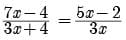21x2 - 12x = 15x2 + 20x - 6x - 8x

6x2 - 26x + 8 = 0

3x2 - 13x + 4 = 0

3x2 - 12x - x + 4 = 0

3x(x - 4) - 1(x - 4) = 0

(x - 4) (3x - 1) = 0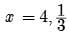So, A is incorrect but R is correct.

Assertion & Reason Test: Triangles - 2 - Question 8

Direction: In the following questions, A statement of Assertion (A) is followed by a statement of Reason (R). Mark the correct choice as.

Assertion : If a line intersects sides AB and AC of a Δ ABC at D and E respectively and is parallel to BC, then AD/AB = AE/AC

Reason : If a line is parallel to one side of a triangle then it divides the other two sides in the same ratio.

Detailed Solution for Assertion & Reason Test: Triangles - 2 - Question 8
We know that If a line is parallel to one side of a triangle then it divides the other two sides in the same ratio. This is Basic Proportionality theorem.

So, Reason is correct.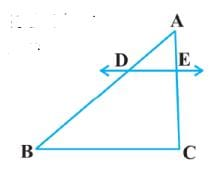By Basic Proportionality theorem, we have AD/DB = AE/EC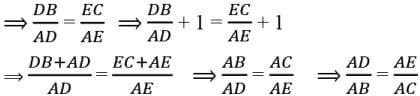So, Assertion is correct

Assertion & Reason Test: Triangles - 2 - Question 9

Direction: In the following questions, A statement of Assertion (A) is followed by a statement of Reason (R). Mark the correct choice as.

Assertion : Assertion : If in a ∆ABC , a line DE || BC , intersects AB in D and AC in E , then AB/AD = AC/AE

Reason : If a line is drawn parallel to one side of a triangle intersecting the other two sides, then the other two sides are divided in the same ratio.

Detailed Solution for Assertion & Reason Test: Triangles - 2 - Question 9
Reason is true : [This is Thale’s Theorem]

For Assertion Since, DE || BC by Thale’s Theorem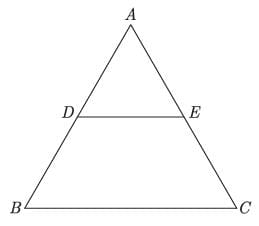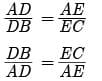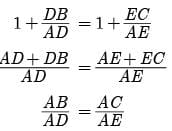Assertion (a) is true

Since, reason gives Assertion.

Assertion & Reason Test: Triangles - 2 - Question 10

Direction: In the following questions, A statement of Assertion (A) is followed by a statement of Reason (R). Mark the correct choice as.

Assertion : ΔABC ~ ΔDEF such that ar(ΔABC) = 36cm2 and ar(ΔDEF) = 49cm2. Then, the ratio of their corresponding sides is 6 : 7

Reason : The ratio of the areas of two similar triangles is equal to the square of the ratio of their corresponding sides.

Detailed Solution for Assertion & Reason Test: Triangles - 2 - Question 10
We know that the ratio of the areas of two similar triangles is equal to the square of the ratio of their corresponding sides.

So, Reason is correct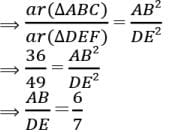So, Assertion is correct.

## Mathematics (Maths) Class 10

115 videos|478 docs|129 tests
Information about Assertion & Reason Test: Triangles - 2 Page
In this test you can find the Exam questions for Assertion & Reason Test: Triangles - 2 solved & explained in the simplest way possible. Besides giving Questions and answers for Assertion & Reason Test: Triangles - 2, EduRev gives you an ample number of Online tests for practice

## Mathematics (Maths) Class 10

115 videos|478 docs|129 tests